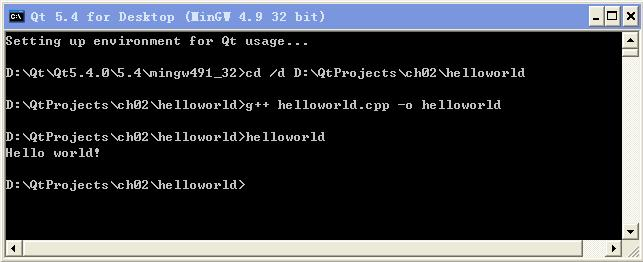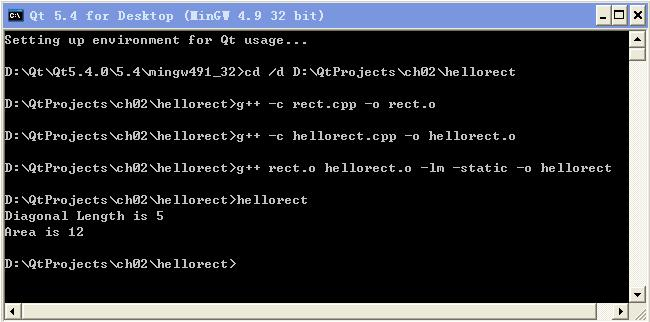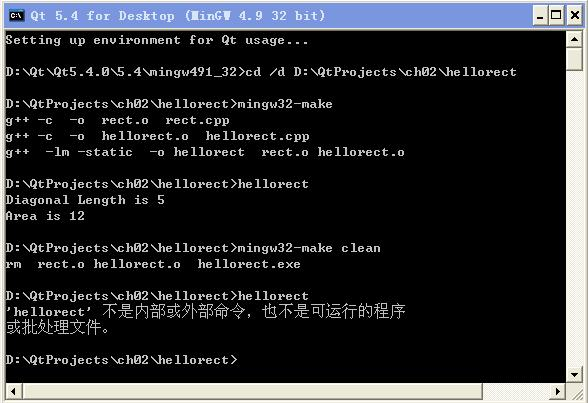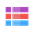2.1 Hello World

2.1.1 简单的 Hello World

//helloworld.cpp
`#include <iostream>`
`using namespace std;`

`int main(int argc, char **argv)`
`{`
`    cout<<"Hello world!"<<endl;`
`    return 0;`
`}`

cd /d D:\QtProjects\ch02\helloworld
g++ helloworld.cpp -o helloworld

helloworld
Linux 系统里面执行当前文件夹的可执行程序，其命令为
./helloworld
Linux 系统管理比较严格，默认不从当前文件夹找可执行程序执行， 所以要加上 ./ 表示从当前文件夹找可执行程序运行。g++ helloworld.cpp -static -o hellostatic

g++ 默认情况下生成的都是 Release 版可执行程序， 如果希望在可执行程序里加入 Debug 调试信息以供 gdb 调试，那需要在 g++ 编译时加一个 -g 选项，比如
g++ helloworld.cpp -g -static -o hellodebug

2.1.2 复杂一些的 Hello Rect

//rect.h
`class Rect`
`{`
`private:`
`    double m_dblWidth;`
`    double m_dblHeight;`

`public:`
`    Rect(double dblWidth, double dblHeight);`
`    double GetWidth(){ return m_dblWidth; }`
`    double GetHeight(){ return m_dblHeight; }`
`    //Functions`
`    double CalcDiagonal();`
`    double CalcArea();`
`};`

//rect.cpp
`#include <cmath>`
`#include "rect.h"`
`using namespace std;`

`Rect::Rect(double dblWidth, double dblHeight)`
`{`
`    m_dblWidth = abs(dblWidth);`
`    m_dblHeight = abs(dblHeight);`
`}`

`double Rect::CalcDiagonal()`
`{`
`    return sqrt(m_dblWidth*m_dblWidth + m_dblHeight*m_dblHeight);`
`}`

`double Rect::CalcArea()`
`{`
`    return m_dblWidth * m_dblHeight;`
`}`

//hellorect.cpp
`#include <iostream>`
`#include "rect.h"`
`using namespace std;`

`int main(int argc, char **argv)`
`{`
`    Rect arect(3.0, 4.0);`
`    cout<<"Diagonal Length is "<<arect.CalcDiagonal()<<endl;`
`    cout<<"Area is "<<arect.CalcArea()<<endl;`
`    return 0;`
`}`

g++ -c rect.cpp -o rect.o
g++ -c hellorect.cpp -o hellorect.o
g++ -c 的意思是编译生成目标代码文件 *.o，而不进行链接。 链接 *.o 文件和链接库生成可执行程序的命令为：
g++ rect.o hellorect.o -lm -static -o hellorect
g++ 会自动调用链接器链接 .o 文件和系统里的链接库， -lm 是链接数学库， -static 是生成静态链接的程序，-o 是指定生成的输出文件名。然后执行 hellorect 就可以看到结果：2.1.3 给 Hello Rect 编写简单的 Makefile

Makefile 文件可以自己编写，其实绝大多数的集成开发环境都可以根据项目文件自动生成相应的 Makefile，所以实际中很多都是集成开发环境自动完成的。这里示范一个简单的 Makefile， 体会一下生成器 make 是如何工作的。

# Makefile for building: hellorect
`CC          = gcc`
`CXX         = g++`
`LINKER      = g++`
`LFLAGS      = -lm -static`

`OBJECTS     = rect.o hellorect.o`
`DSTTARGET   = hellorect`
`# Default rule`
`all: \$(DSTTARGET)`

`\$(DSTTARGET): \$(OBJECTS)`
`	\$(LINKER)  \$(LFLAGS)  -o \$@  \$(OBJECTS)`

`hellorect.o: hellorect.cpp`
`	\$(CXX) -c  -o  \$@  \$<  `

`rect.o: rect.cpp`
`	\$(CXX) -c  -o  \$@  \$<  `

`clean:`
`	rm  \$(OBJECTS)  hellorect.exe`

[tab字符] 系统命令

all: \$(DSTTARGET)

\$(DSTTARGET): \$(OBJECTS)

`	\$(LINKER)  \$(LFLAGS)  -o \$@  \$(OBJECTS)`

`hellorect.o: hellorect.cpp      `
`	\$(CXX) -c  -o  \$@  \$<  `

`rect.o: rect.cpp`
`	\$(CXX) -c  -o  \$@  \$<  `

`clean:`
`	rm  \$(OBJECTS)  hellorect.exe`
rm 是删除命令，如果 Windows 系统里没有 rm 命令，请安装一个 msysgit 工具（ http://msysgit.github.io/）， 然后系统环境变量里面会有 msysgit 工具路径，里面有 rm 工具。 clean 做的事情就是删除项目生成的 .o 和 .exe 文件。（注：Linux 系统里可执行程序没有 .exe 后缀，需要去掉 .exe 后缀。）

mingw32-make

mingw32-make clean
MinGW 里面是用 mingw32-make 生成程序。（注：Linux 系统里直接用 make 。） 在 MinGW 环境的命令示范如下：